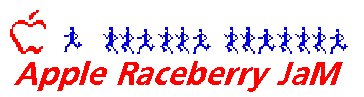# Run Minnesota 6XMile Como Park Relay

## Aug. 11, 2021

81 degrees
Results by John Cramer

```                                 Last updated Aug. 17, 2021 12:15 PM

PLACE         FINISHER          TIME

Men's Division

1. 1
1  Muhammed Bati              31:50
33  Muhammed Bati
Total Time = 31:50     Total Places = 1

2. 2
2  Daniel Docherty            32:20
Total Time = 32:20     Total Places = 2

3. 3
3  Tyler Merrell              33:33
35  Felix Ongara
Total Time = 33:33     Total Places = 3

4. 4
4  Dan Gerber                 34:10
36  Victor Sanchez
Total Time = 34:10     Total Places = 4

5. 5
5  Dallas White               34:19
37  Brandan Kotek
Total Time = 34:19     Total Places = 5

6. 6
6  Kevin Torstenson           34:45
38  Samuel Lindner
Total Time = 34:45     Total Places = 6

7. 7
7  Freddie                    35:15
39  Eddie McCorkle
Total Time = 35:15     Total Places = 7

8. 8
8  Christian Leitner          35:48
40  Tyler Karl
Total Time = 35:48     Total Places = 8

9. 9
9  Patrick Nelson             35:54
41  Dan Luoma
Total Time = 35:54     Total Places = 9

10. 10
10  Mason Aarness              36:23
42  Charlie Power-Thiesen
Total Time = 36:23     Total Places = 10

11. 11
11  Chris Gill                 36:26
43  Cole Konczal
Total Time = 36:26     Total Places = 11

12. 12
12  Antero Simla               36:36
44  Jackson Lee
Total Time = 36:36     Total Places = 12

13. 13
13  Matt Haechrel              37:34
45  Drew Carlson
Total Time = 37:34     Total Places = 13

14*. 14
14  Michies Kiros              38:23
Total Time = 38:23     Total Places = 14

15. 18
16  Naci Konar-Steenberg       39:46
48  Edward Gronau
Total Time = 39:46     Total Places = 16

16. 25
17  Peter Kessler              41:35
49  Bill Dobbs
Total Time = 41:35     Total Places = 17

17. 28
18  Kaj                        42:36
50  Andy Sackreiter
Total Time = 42:36     Total Places = 18

18. 29
19  Stephen Hays               42:37
51  Steven Ruach
Total Time = 42:37     Total Places = 19

19. 30
20  Kiel McGlum                42:40
52  Peter O'Yamashiro
Total Time = 42:40     Total Places = 20

20. 31
21  Nate                       42:50
53  Liam Parlin
Total Time = 42:50     Total Places = 21

21. 36
22  Chris Thoemke              45:24
54  Kirt Goetzke
Total Time = 45:24     Total Places = 22

22. 41
23  Jarred                     50:29
55  Owen Sampson
Total Time = 50:29     Total Places = 23

23. 42
24  Atticus Bird               51:35
56  Wyatt Deimel
Total Time = 51:35     Total Places = 24

24. 43
25  Bennett Deimel             52:30
57  Sunnatillo Mammatkulov
Total Time = 52:30     Total Places = 25

25. 48
26  John Magnuson              55:48
58  John Nasland
Total Time = 55:48     Total Places = 26

26. 49
27  John Arnett                56:10
59  Tom Couillard
Total Time = 56:10     Total Places = 27

27. 50
28  Eric Hanson                56:50
60  Joe Gronau
Total Time = 56:50     Total Places = 28

28. 53
29  Magnus Halvonson           59:32
61  Liam Doyle
Total Time = 59:32     Total Places = 29

29. 17
15  Doug                       59:33
47  Alex Cowles
Total Time = 59:33     Total Places = 15

30. 54
30  Jim Smieja                 59:42
62  Howard Ojalvo
Total Time = 59:42     Total Places = 30

31. 55
63  Bennett Ludwig
Total Time = 1:00:25     Total Places = 31

32. 56
32  Henry Richardson         1:01:31
64  Max Johnson
Total Time = 1:01:31     Total Places = 32

PLACE         FINISHER          TIME

Women's Division

1. 59
1  Rhynn Paulson              39:30
9  Emmy Farago
Total Time = 39:30     Total Places = 33

2. 16
2  Quinn O'Connor             39:31
10  Alicia Belany
Total Time = 39:31     Total Places = 34

3. 19
3  Gabrielle Bolcer           40:08
11  Lauren Ellefson
Total Time = 40:08     Total Places = 35

4. 35
4  Ellie Frosig               44:30
12  Mack Keller-Miller
Total Time = 44:30     Total Places = 36

5. 40
5  Meg Gronau                 49:52
13  Sar Gable
Total Time = 49:52     Total Places = 37

6. 44
14  Rebecca Deimel
Total Time = 53:27     Total Places = 38

7. 45
7  Keegan Eng                 53:37
15  Tina Tram
Total Time = 53:37     Total Places = 39

8. 46
8  Jan Kihm                   54:27
16  Joanna Rameriz
Total Time = 54:27     Total Places = 40

PLACE         FINISHER          TIME

Coed Division

1. 15
1  James Brand                38:23
19  Nicole Cueno
Total Time = 38:23     Total Places = 41

2. 20
2  Maleta                     40:28
20  Mike Moulsoff
Total Time = 40:28     Total Places = 42

3*. 21
3  Kellen Overvig             40:33
Total Time = 40:33     Total Places = 43

3*. 23
5  Sam Karlson                40:33
23  Martha Hubbell
Total Time = 40:33     Total Places = 45

5. 22
4  Daisy Richmond             40:48
22  Jesse Longley
Total Time = 40:48     Total Places = 44

6. 24
6  Luke Gesior                41:15
24  Jen Rolfes
Total Time = 41:15     Total Places = 46

7. 26
7  Maene Lindson              41:43
Total Time = 41:43     Total Places = 47

8. 27
8  Anthony                    42:02
26  Alona Schamm
Total Time = 42:02     Total Places = 48

9. 32
9  Sarah Barker               43:03
27  Noah Blenkmore
Total Time = 43:03     Total Places = 49

10. 33
10  Andy                       43:23
28  Audrey Schwen
Total Time = 43:23     Total Places = 50

11. 34
11  Emily                      44:23
29  Justin Jones
Total Time = 44:23     Total Places = 51

12. 37
12  Ellen Ryan                 46:36
30  David Petrich
Total Time = 46:36     Total Places = 52

13. 38
13  Libby                      47:14
31  Matt Johnson
Total Time = 47:14     Total Places = 53

14. 39
14  Matt Olson                 47:55
32  Andrea McKennan
Total Time = 47:55     Total Places = 54

15. 47
15  Michael                    55:43
33  Alicyn Bolland
Total Time = 55:43     Total Places = 55

16. 51
16  Ida Thoreen                58:14
34  Logan Kittok
Total Time = 58:14     Total Places = 56

17. 52
17  Alaina Orsted              59:28
35  Brodan Behling
Total Time = 59:28     Total Places = 57

18. 57
18  Don Soule                1:15:27
36  Marcy Hokenson
Total Time = 1:15:27     Total Places = 58

```